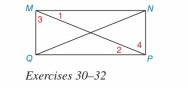Chapter 3.2, Problem 31E### Elementary Geometry for College St...

6th Edition
Daniel C. Alexander + 1 other
ISBN: 9781285195698

#### Solutions

Chapter
Section### Elementary Geometry for College St...

6th Edition
Daniel C. Alexander + 1 other
ISBN: 9781285195698
Textbook Problem
1 views

# In Exercises 30 to 32, draw the triangles that are to be shown congruent separately. Then complete the proof.Given: ∠ 1 ≅ ∠ 2 and M N ¯ ≅ Q P ¯ Prove: M Q ¯ ∥ N P ¯ (HINT: Show Δ N M P ≅ Δ Q P M .)

To determine

To prove:

The given statement 12 and MN¯QP¯.

Explanation

Given:

The given statement is,

12 and MN¯QP¯

Figure (1)

Properties used:

(1) If two lines are cut by a transversal so that two corresponding angles are congruent, then these lines are parallel.

(2) In two triangles, if two corresponding sides and the included angle between them is congruent, then the triangles are congruent.

(3) If two triangles are congruent to each other then all angles of the triangle are congruent to each other.

Approach:

The required triangles are,

Figure (2)

The given statements are,

12 and MN¯QP¯

The side MP is common in both triangles ΔNMP and ΔQMP.

So,

MP¯MP¯

Use SAS criteria of triangle congruency.

ΔNMPΔQMP

Use transitive property of congruence.

24

2 and 4 are congruent corresponding angles.

Thus, MQ¯NP¯.

 PROOF Statements Reasons 1. MN¯≅QP¯ 1. Given 2.MP¯≅MP¯ 2

### Still sussing out bartleby?

Check out a sample textbook solution.

See a sample solution

#### The Solution to Your Study Problems

Bartleby provides explanations to thousands of textbook problems written by our experts, many with advanced degrees!

Get Started

#### Find more solutions based on key concepts